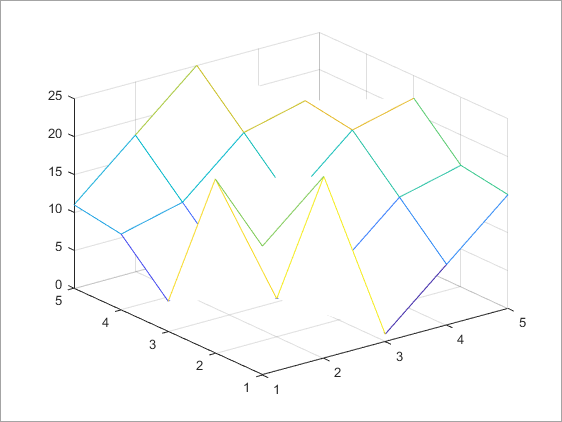# eval

Evaluate MATLAB expression

## Syntax

``eval(expression)``
``[output1,...,outputN] = eval(expression)``

## Description

example

````eval(expression)` evaluates the MATLAB® code in `expression`. NoteIn most cases, using the `eval` function is less efficient than using other MATLAB functions and language constructs, and the resulting code can be more difficult to read and debug. For more information, see Alternatives to the eval Function. ```

example

````[output1,...,outputN] = eval(expression)` returns the outputs from `expression` in the specified variables.```

## Examples

collapse all

Use `eval` to evaluate and plot the expression `magic(5)`.

```Z = eval('magic(5)'); mesh(Z)```## Input Arguments

collapse all

Expression to evaluate, specified as a character vector or string scalar. `expression` must be a valid MATLAB expression and must not include any MATLAB keywords. To determine whether a word is a MATLAB keyword, use the `iskeyword` function.

Example: `eval('magic(5)')`

## Output Arguments

collapse all

Outputs from evaluated expression, returned as any MATLAB data type.

## Limitations

• If you use `eval` within an anonymous function, nested function, or function that contains a nested function, the evaluated `expression` does not create any variables.

## Tips

• To allow the MATLAB parser to perform stricter checks on your code and avoid untrapped errors and other unexpected behaviors, do not include output arguments in the input to the `eval` function. For example, the statement `eval(['output = ',expression])` is not recommended.

Instead, specify output arguments to the `eval` function to store the results of the evaluated expression. For example:

` output = eval(expression)`